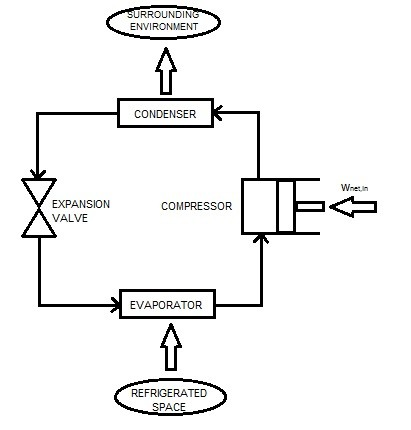# Refrigerators & Heat Pumps

Heat naturally transfers in the direction of decreasing temperature.  This means that the energy from a higher temperature medium will flow to an object with a lower temperature.  Refrigerators and heat pumps take advantage of this fact.

### Refrigerators

First, let’s take a look at refrigerators.  Refrigerators are devices that have the capability of moving heat from a low temperature medium to a higher temperature medium.  At first you might say this is impossible since it goes against the second law.  However, a refrigerator makes this possible by using a working fluid called a refrigerant.  The refrigerant moves the heat between two different environments.

Like a heat engine, refrigerators are cyclic devices.   The vapor-compression refrigeration cycle is the most common cycle.   This cycle requires the use of four main components, a compressor, a condenser, an expansion valve , and an evaporator.  Refer to the figure below for a basic diagram.Before the refrigerant reaches the expansion valve it will be in a high pressure liquid state. When it enters the  expansion valve  its pressure will drop drastically.  In turn, this will cause a large temperature  and pressure drop.  The temperature drop will be lower than the refrigerated space.  Next it will enter the evaporator coils.  In these coils the refrigerant will absorb heat from the refrigerated space.  As this occurs the refrigerant will evaporate.  Next, the compressor will increase both the pressure and temperature of the refrigerant.  The temperature of the refrigerant will be greater than the surrounding enthronement.  Finally, the refrigerant will pass through the condenser coils allowing the energy to transfer to the outside environment.  This will cause the refrigerant to return to a liquid state.  Finally, the cycle will than repeat.

### Refrigerator Coefficient of Performance

By the second law of thermodynamics a cycle cannot be 100% efficient.  To run the compressor work is required.   As a result, the additional work energy will prevent the cycle from being 100 % efficient, which will satisfy the second law of thermodynamics.  For a refrigerator, the efficiency is determined by the coefficient of performance, $COP_R$.  The following equation is used to calculate the $COP_R$.

(Eq 1)   $COP_R=\frac{Q_L}{W_{NET,in}}=\frac{Q_L}{Q_H-Q_L}=\frac{1}{Q_H/Q_L-1}$

Unlike efficiency, $COP_R$ can be greater than one.  This is because it compares the amount of heat removed from the refrigerator in respect to the input work.

### Heat Pump

A heat pump, like a refrigerator, transfers heat from a low temperature environment to a high temperature environment.   As a result, a heat pump will operate on the same cycle as a refrigerator.  However, the objective of a heat pump is different in comparison to a refrigerator.  A refrigerator is designed to maintain a refrigerated space at a low temperature.  On the other hand, a heat pumps objective is to maintain a heated space at a high temperature.  To do this a heat pump must be able to absorb heat from a low temperature source.  This source could be water, the cold air outside, or the ground.  After absorbing the heat it will than be moved inside to heat the heated space.  The diagram below shows a heat pumps cycle.### Heat Pump Coefficient of Performance

Just like a refrigerator a heat pump will also have a coefficient of performance.  In order to calculate the $COP_{HP}$, you will need to use the equation below.

(Eq 2) $COP_{HP}=\frac{Q_H}{Q_H-Q_L}=\frac{1}{1-Q_L/Q_H}$

In addition, the $COP$ of a heat pump and refrigerator can be related to each other using the following equation.